# How to use the SUMIFS function in Excel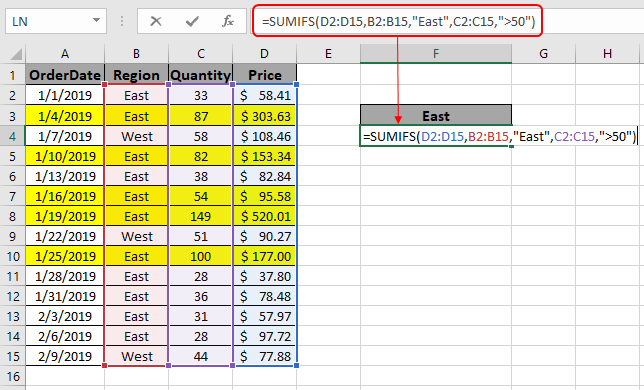In this article, we will learn How to use the SUMIFS function in Excel.

Sum if with criteria

In simple words, while working with a long data sheet. Sometimes we need to find the sum of values having multiple criteria. SUMIFS function formula finds the SUM of the array where multiple conditions need to be satisfied. Let's learn the SUMIFS function Syntax and an example to illustrate the result.

SUMIFS Function in Excel

SUMIFS function returns the sum of range having multiple criterias.

Syntax of formula:

 = SUMIFS ( Sum_range , range1 , "value1" , range2, ">value2", ….)

Sum_range : range where sum is required

range1 : range where criteria value1 is applied

Value1 : value criteria applied on range1

range2 : range where criteria value2 is applied

Value2 : value criteria applied on range2

Example :

All of these might be confusing to understand. Let's understand how to use the function using an example.

Here we have a list of received orders and their respective values.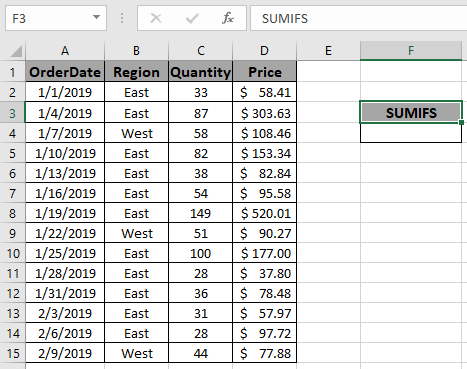Here we need to find the TotalPrice sum if the region is EAST and Quantity is greater than 50.

So we will use the formula to get the total amount

 = SUMIFS ( D2:D15 , B2:B15 , "East" , C2:C15 , ">50" )

D2:D15 : Sum_range where values gets sum

B2:B15 :  range where criteria value1 is applied.

"East" : value criteria applied on range1.

C2:C15 : range where criteria value2 is applied.

">50" : value criteria applied on range2.

Explanation for the formula:

Here the range B2:B15 checks for the value East (Region) and in range C2:C15 catches all the quantity greater than 50. Then the function finds out the sum from the sum_range (D2:D15) taking the values satisfying the both conditions.Here the arguments to the function is given as cell reference.

Use the formula as stated above to calculate the total price and click Enter.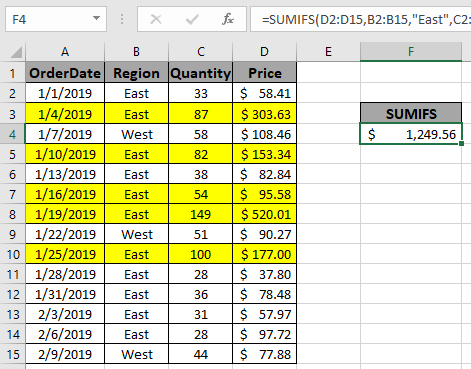As you can see the formula returns \$1249.56 , the Total price for the yellow rows marked.

SUMIFS with date values

Syntax:

 =SUMIFS(Sum_range , range ,">=" & date1, range, "<=" & date2)

Sum_range : range where sum is required

range : Set of dates

& : operator used to concatenate other ope

Example

Here we need to find the TotalPrice sum if date is between 2/20/2019 (after) & 7/9/2019 (before).So we will use the formula to get the amount

 =SUMIFS (F2:F10 , A2:A10 , ">=" & I3, A2:A10 , "<=" & J3)

F2:F10 : Sum_range

A2:A10 : range where condition is applied

">=" & I3 : greater than date value in I3(2/20/2019).

"<=" & J3 : less than date value in J3.(7/9/2019).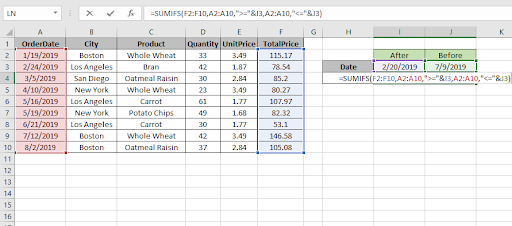Use the formula as stated above and click Enter.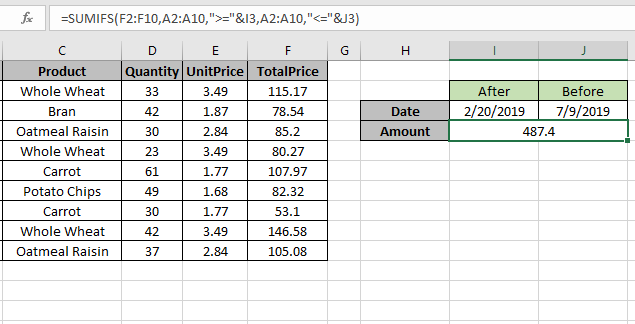As you can see the formula returns 487.4, the Totalprice between dates.

For customization just change the dates in I3 & J3 cell and get the results with the formula.

Here are some observational results using the SUMIFS function in Excel.

Notes:

1. The SUMIFS function supports logical operators like <, >, <>, = but these are used using double quote sign ( " ) .
2. The SUMIFS function also supports Wildcards ( * , ? ) which helps in extracting values having phrases.
3. Non - numeric values must be provided in double quotes ("value").
4. The function returns the sum of the values satisfying all the conditions.

Hope this article about How to use the SUMIFS function in Excel is explanatory. Find more articles on calculating values and related Excel formulas here. If you liked our blogs, share it with your friends on Facebook. And also you can follow us on Twitter and Facebook. We would love to hear from you, do let us know how we can improve, complement or innovate our work and make it better for you. Write to us at info@exceltip.com.

Related Articles :

How to use the SUMPRODUCT function in Excel: Returns the SUM after multiplication of values in multiple arrays in excel.

SUM if date is between : Returns the SUM of values between given dates or period in excel.

Sum if date is greater than given date: Returns the SUM of values after the given date or period in excel.

2 Ways to Sum by Month in Excel: Returns the SUM of values within a given specific month in excel.

How to Sum Multiple Columns with Condition: Returns the SUM of values across multiple columns having condition in excel

How to use wildcards in excel : Count cells matching phrases using the wildcards in excel

Popular Articles :

How to use the IF Function in Excel : The IF statement in Excel checks the condition and returns a specific value if the condition is TRUE or returns another specific value if FALSE.

How to use the VLOOKUP Function in Excel : This is one of the most used and popular functions of excel that is used to lookup value from different ranges and sheets.

How to use the SUMIF Function in Excel : This is another dashboard essential function. This helps you sum up values on specific conditions.

How to use the COUNTIF Function in Excel : Count values with conditions using this amazing function. You don't need to filter your data to count specific values. Countif function is essential to prepare your dashboard.

Terms and Conditions of use

The applications/code on this site are distributed as is and without warranties or liability. In no event shall the owner of the copyrights, or the authors of the applications/code be liable for any loss of profit, any problems or any damage resulting from the use or evaluation of the applications/code.